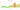# ฿

## Thai Baht

Thailand's national currency is the baht. There are 100 satang in it. The Bank of Thailand is in charge of currency production. As of January 2019, SWIFT classified the Thai baht as the tenth-most popular currency for international payments.

#### how has the value of the currency changed in the last year?## USD 1 = THB 36.577

How much is10 US dollars worth inThai baht?
At the current exchange rate, 10 US dollars is worth 365.77 Thai baht
How much is50 US dollars worth inThai baht?
At the current exchange rate, 50 US dollars is worth 1,828.83 Thai baht
How much is100 US dollars worth inThai baht?
At the current exchange rate, 100 US dollars is worth 3,657.65 Thai baht
How much is500 US dollars worth inThai baht?
At the current exchange rate, 500 US dollars is worth 18,288.25 Thai baht
How much is2,000 US dollars worth inThai baht?
At the current exchange rate, 2,000 US dollars is worth 73,153 Thai baht
How much is10 US dollars worth inThai baht?
At the current exchange rate, 10 US dollars is worth 365.77 Thai baht
How much is50 US dollars worth inThai baht?
At the current exchange rate, 50 US dollars is worth 1,828.83 Thai baht
How much is100 US dollars worth inThai baht?
At the current exchange rate, 100 US dollars is worth 3,657.65 Thai baht
How much is500 US dollars worth inThai baht?
At the current exchange rate, 500 US dollars is worth 18,288.25 Thai baht
How much is2,000 US dollars worth inThai baht?
At the current exchange rate, 2,000 US dollars is worth 73,153 Thai baht